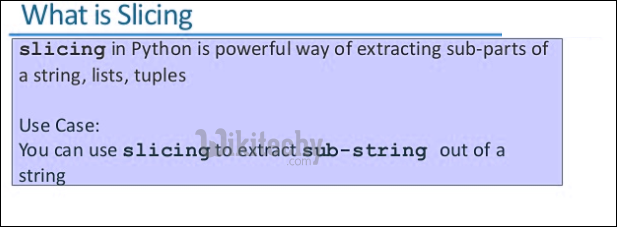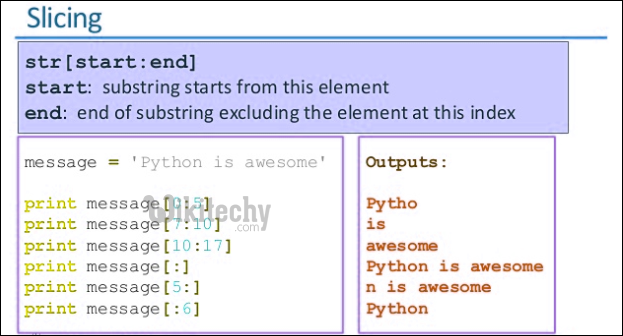# python tutorial - String Python | Interesting facts about strings in Python | Slicing - learn python - python programmingLearn Python - Python tutorial - interesting-facts-about-strings-in-python - Python examples - Python programs

Like other programming languages, it’s possible to access individual characters of a string by using array-like indexing syntax. In this we can access each and every element of string through their index number and the indexing starts from 0. Python does index out of bound checking.So, we can obtain the required character using syntax, string_name[index_position]:

• The positive index_position denotes the element from the starting(0) and the negative index shows the index from the end(-1).

Example-

python - Sample - python code :

python tutorial - Output :

python - Sample - python code :

Slicing

To extract substring from the whole string then then we use the syntax likepython - Sample - python code :• beginning represents the starting index of string
• end denotes the end index of string which is not inclusive
• steps denotes the distance between the two words.

Note: We can also slice the string using beginning and only and steps are optional.

Example-

python - Sample - python code :

python tutorial - Output :

Wikitechy tutorial site provides you all the learn python , python course for beginners , learn python online interactive , how to learn python coding , best online python training , python online course certification free , python training for beginners , online python course free , learn python online free course